Study S4 Mathematics Probability of Combined Events - Geniebook# Probability of Combined Events

In this particular article we will be learning about the Probability of Combined Events. We will learn about

• Probability of simple combined events
• Possibility diagrams

## Introduction

Probability refers to the measure of chances. In probability, the sample space refers to the possible results of an experiment.

### Example 1:

A fair die is rolled.

Find the probability that the number shown is

1. Odd,
2. A multiple of 3

Solution:

1. The total numbers of Sample Space are: (1, 2, 3, 4, 5, 6)

In the sample space there are 3 odd numbers. There are 6 possibilities of outcomes.

So,

The probability to get an odd outcome is:

P (Odd)\begin{align*} &=\frac{3}{6} \end{align*}

\begin{align*} =\frac{1}{2} \end{align*}

1. Multiple of 3 is 3 and 6.

There are only 2 numbers that are multiples of 3.

Therefore, the probability of choosing a number that is a multiple of 3 is:

P (multiple of 3)\begin{align*} &=\frac{2}{6} \end{align*}

\begin{align*} &=\frac{1}{3} \end{align*}

Example 2:

A bag contains 6 red balls, 4 blue balls, and 8 green balls. A ball is chosen at random and not replaced. Find the probability that the ball drawn is

1. Blue
2. Not green

Solution:

There are 6 red balls, 4 blue balls and 8 green balls.

So, the total number of balls is:

$$6+4+8= 18$$ balls.

1.

\begin{align*} \text {Probability Of Blue Balls} &= \frac {\text {Total Number Of Blue Balls}} {\text {Total Number Of Balls}} \\ \\ &= \frac {4}{18} \\ \\ &= \frac {2}{9} \end{align*}

This means that when a ball is randomly taken out from the total 18 balls, the probability of getting a blue ball is $$2\over9$$.

1. Method 1:

To get a ball that is not green:

\begin{align*} \text {Probability (Not Green)} &= \frac {\text {(Total Number Of Red Balls + Total Number Of Blue Balls)}} {\text {Total Number Of Balls}} \\ \\ &=\frac{(6+4)}{18} \\ \\ &= \frac{10}{18} \\ \\ &= \frac{5}{9} \end{align*}

Method 2:

The probability of getting Red/ Blue/ Green Balls is 100%. 100% is considered as 1 (whole). So, 1 represents all the possibilities of getting the total number of balls.

\begin{align*} \text {Probability (not green)} &= \text {1}- \text{(Probability Of Getting Green Balls) } \\ \\ &= 1-\frac {8}{18} \\ \\ &= \frac {5}{9} \end{align*}

## Possibility Diagrams

What are the possible outcomes of rolling just one fair die?

The total number of outcomes are:

 1 2 3 4 5 6

The total number of outcomes is 6 outcomes.

What are the possible outcomes of rolling two fair dice?

 (1,1) (1, 2) (1,3) (1,4) (1,5) (1,6) (2,1) (2,2) (2,3) (2,4) (2,5) (2,6) (3,1) (2,3) (3,3) (3,4) (3,5) (3,6) (4,1) (2,4) (4,3) (4,4) (4,5) (4,6) (5,1) (2,5) (5,3) (5,4) (5,5) (5,6) (6,1) (6,2) (6,3) (6,4) (6,5) (6,6)

When two fair dice are rolled, the total number of outcomes are 36. The above table shows all the 36 possible outcomes when two fair dice are rolled. The total 36 outcomes are obtained by:

$$\text {6 (Total Outcomes Of 1st Die)} × \text{6 (Total Outcomes Of 2nd Die)} = 36$$

So, how do we put these outcomes in a systematic table?

By drawing an example of a possibility diagram,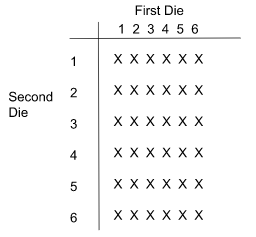In the table, the horizontal column has possible outcomes of First Die. The vertical column has possible outcomes of Second Die. For each combination, a cross shows the number of possible outcomes. Therefore, the number of crosses is 36, which shows the possible outcomes of rolling two fair dice.

### Example 3:

Two fair dice are thrown at different times. Find the probability that

1. Both dice show the same number,
2. The number shown by one of the dice is 1 and the number shown by the other dice is not 1,
3. The number shown on the first dice is 4 and the number shown on the second dice is odd.

Solution:

1. In the below possibility diagram, the horizontal column has possible outcomes of the 1st die and the vertical column has possible outcomes of the 2nd die.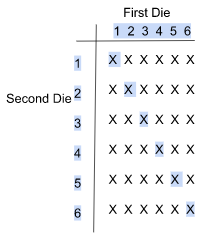\begin{align*} \text {The total possible outcomes, when both dice show the same number are}= 6. \end{align*}

All of them are highlighted in blue.

\begin{align*} \text {Probability (Same Number)} &= \frac {6}{36} \\ \\ &= \frac {1}{6} \end{align*}

1. In the below possibility table, the horizontal column has possible outcomes of the 1st die and the vertical column has possible outcomes of the 2nd die.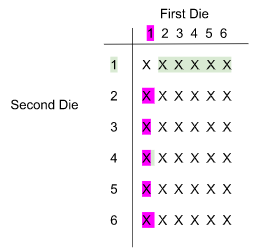\begin{align*} \text {The total possible outcomes when one die is 1 and the other die is not one are}= 10. \end{align*}

All of them are highlighted in green and pink.

\begin{align*} \text {Probability (one die is 1, other die is not 1)} &= \frac {10}{36} \\ \\ &= \frac {5}{18} \end{align*}

1. In the below possibility table, the horizontal column has possible outcomes of 1st die and the vertical column has possible outcomes of 2nd die.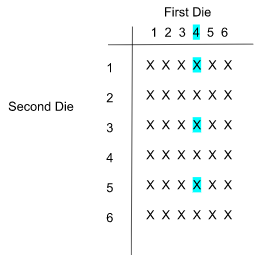The first die is 4. The odd possibility in the second die is 1, 3, 5. All of them are highlighted in green.

So, the total possibility for a second die is 3.

\begin{align*} \text {Probability ( First die is 4, Second die is odd outcome)} &= \frac {3}{36} \\ \\ &= \frac {1}{12} \end{align*}

This is how we use the possibility diagram to answer probability-related questions.

It is very important to note that the above example is about the probability of a combined event. A combined event has two or more variable factors in the experiment.

Example 4:

Deck A contains cards that are numbered 2, 4, 6 and 8.

Deck B contains cards that are numbered 1, 3, 5 and 7.

A card is drawn, at random, from Deck A, followed by Deck B.

Find the probability that the sum of the two numbers shown is

1. Greater than 10,
2. Even

Solution: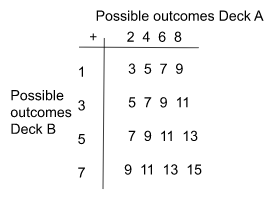In the table, the given possibilities of Deck A and Deck B are represented. The + indicates that the possibilities of Deck A are added to the possibilities of Deck B. Here, the total outcomes are 16.

1. In the below possibility table, the possible outcomes of Deck A and Deck B are represented.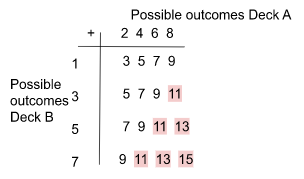The total possible outcomes that are greater than 10 are= 6.

All are highlighted in red.

\begin{align*} \text {Probability (greater than 10)} &= \frac {6}{16} \\ \\ &= \frac {3}{8} \end{align*}

1.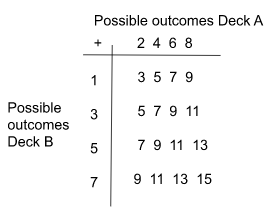None of the outcomes are even. So,

$$\text {Probability (even)} = 0$$

## Conclusion

In this article, we studied the Probability of Combined Events as per the syllabus of Secondary 4 Mathematics class. We understood the concept of probability and how it can be explained through possibility diagrams.

Continue Learning
Sets: Venn Diagrams, Intersections & Union Probability of Combined Events
Statistical Data Analysis Matrices
VectorsPrimaryPrimary 1Primary 2Primary 3Primary 4Primary 5Primary 6SecondarySecondary 1Secondary 2Secondary 3Secondary 4PhysicsChemistryMathsE-Maths
Sets: Venn Diagrams, Intersections & Union
Probability of Combined Events
Statistical Data Analysis
Matrices
VectorsA-Maths